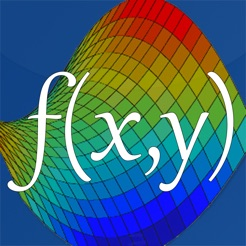## Captures d’écran

•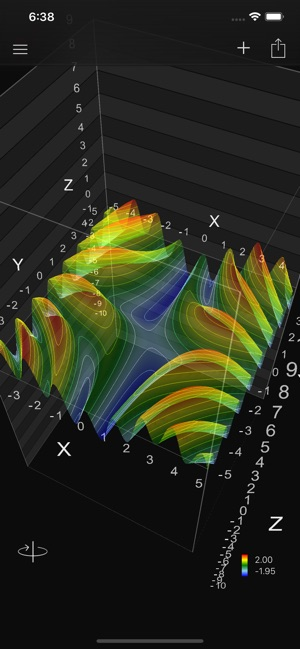•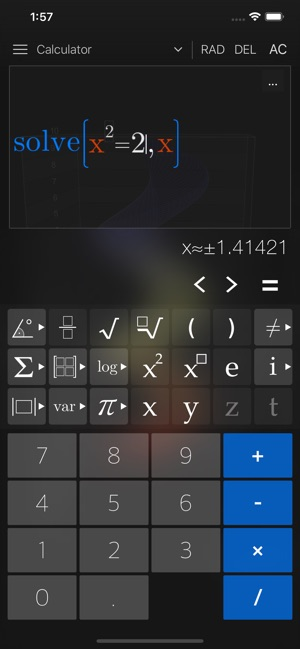•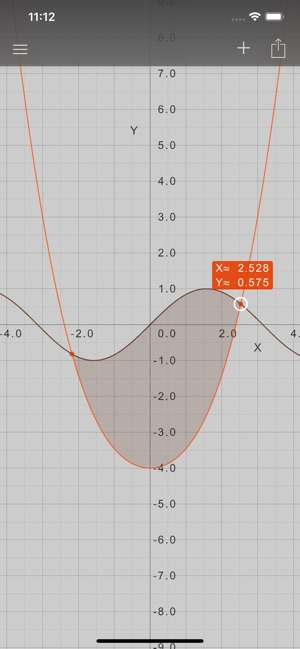•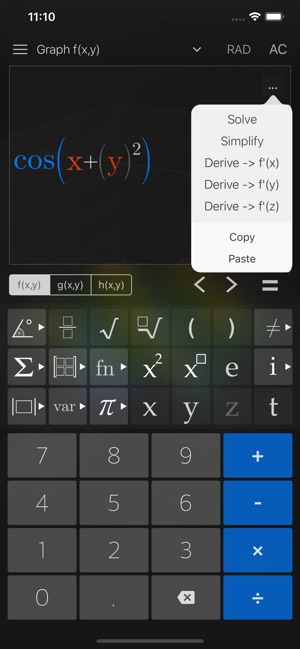••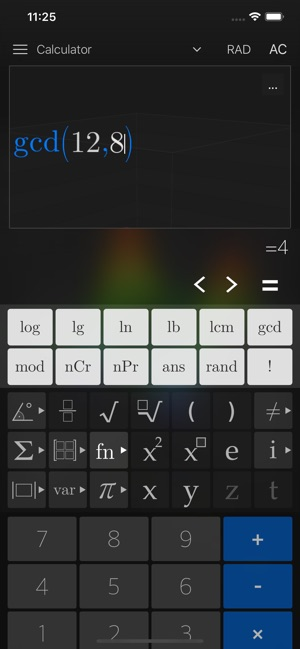•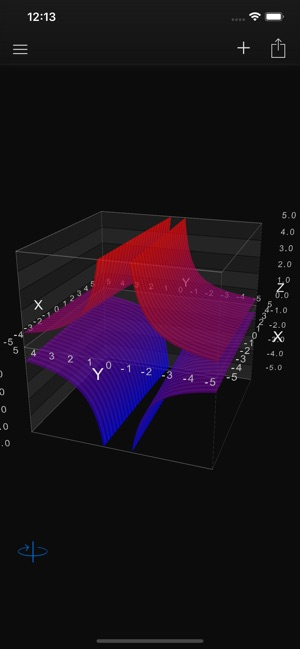•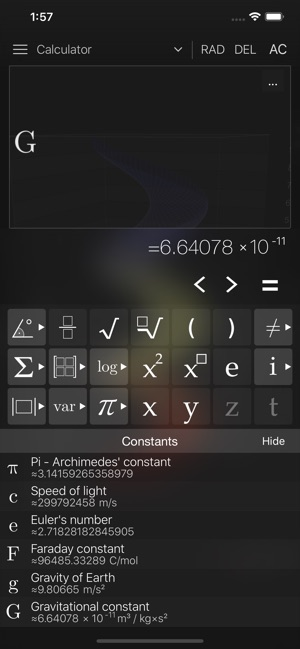•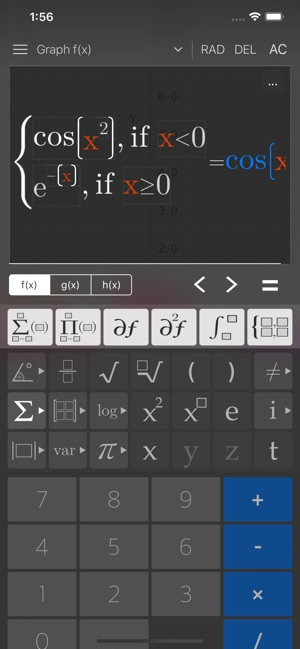•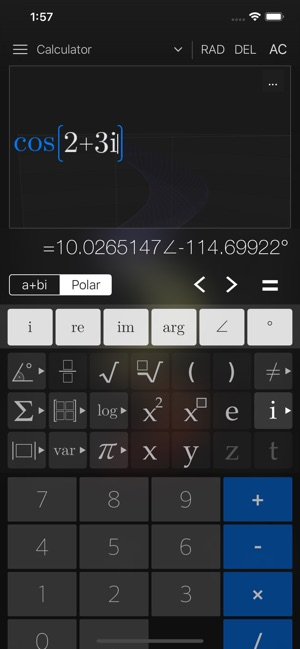## Description

Visual Math 4D is a graphical calculator that allows you to visualize and solve your mathematical equations. It supports various types of equations, such as polar, spherical, parametric and implicit equations, which can be visualized and animated in 2D and 3D. You can also plot and animate vector fields in 2D and 3D.

Features:
- solve equations
- plot cartesian functions with intersections
- plot polar- and spherical functions
- plot parametric equations in 2D and 3D
- plot complex functions (draw real part & imaginary part)
- plot vector fields in 2D and 3D
- plot implicit equations in 2D and 3D
- plot contour maps ins 2D and 3D
- support for complex numbers
- vectors & matrices
- truth- and value tables
- trigonometrical and hyperbolic functions
- piecewise-defined functions
- logarithmic functions
- logical & binary operators
- definite integral
- n-th derivations
- statistical functions (nPr, nCr, rand..)
- physical and mathematical constants with their units
- animate variables
- share content with other apps
- no network connection required
- and a lot more

The app is easy to use and helps students and engineers to visualize and solve their mathematical equations.

## Nouveautés

Version 5.3

- draw intersection points and show their coordinates
- optimized expression rendering
- select and focus functions using gestures.
- new functions are supported, like modulo, fact, nPr, nCr, lcm, gcd, rand etc..
- support for the last result (ans)
- copy & paste your equations
- new device support

## Notes et avis

4.5 sur 5
47 notes

47 notes

jpalluin ,

### Trop de plantages

Beaucoup de bugs, parfois on ne peut plus sortir du mode graphique.
C'était mieux avant.

## Informations

Distributeur
Ronny Weidemann
Taille
117.2 Mo
Catégorie
Éducation
Compatibilité

Nécessite iOS 10.0 ou une version ultérieure. Compatible avec l’iPhone, l’iPad et l’iPod touch.

Langues
Âge
Classé 4+
Prix
2,29 €
Achats intégrés
1. Coffee 1,09 €

## Prend en charge

•